top of page
Search
• Tracy Gunn

# Understanding Archimedes` Principle in Physics IDC Dive Theory

Updated: 4 days agoArchimedes' Principle states that the upward buoyant force that is exerted on a body immersed in a fluid, whether fully or partially submerged, is equal to the weight of the fluid that the body displaces.

## Archimedes of Syracuse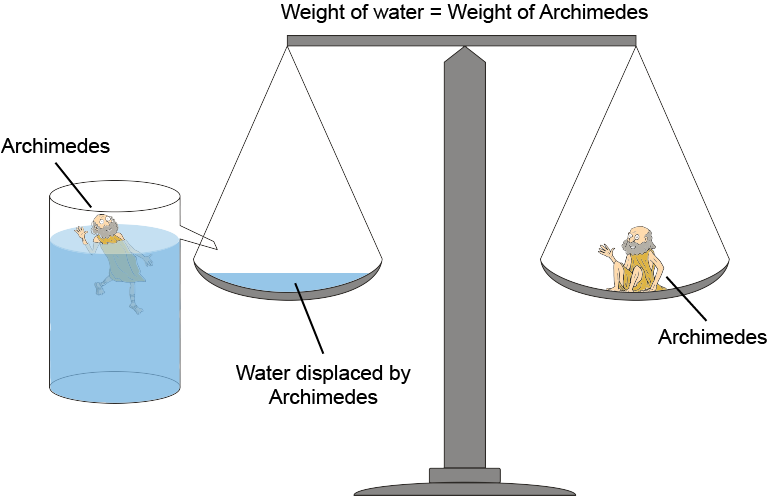Archimedes went down in history for famously running naked down the street of Syracuse yelling “Eureka” when he realized that the water displaced in his bathtub was equal to the weight of his body; thus the Archimedes principle was born (sometime between 287-212 BC). Check out the history here..

## Applications

Archimedes` Principle is used in many applications from measuring volumes of irregular objects (such as crowns in the above history), to explaining behaviors of any object placed in fluids. It lets us know how ships float, submarines dive and hot air balloons fly. The real-life applications are ongoing with many more interesting examples given by Science Clarified.

Many scientific research projects benefit from using Archimedes' Principle such as medical, engineering, entomology, and geology.

It can also be one of the more difficult concepts of diving theory to grasp. Don`t worry, you are not alone, and it is our aim here to help you get comfortable with those tricky Archimedes questions. It gets easier with practice.

* Note. Before we begin it is worthy to mention that litres (and various other words) is/are not misspelled in this blog. I am Australian and that is how we spell it (them).

## Understanding Archimedes` Principle

Let´s start by talking about buoyancy.

You already know that we need a buoyancy check.

• When we change our dive equipment

• When we change our dive environment

• When I haven’t been diving in a while.

And these all relate to changes in buoyancy and Archimedes' Principle if you think about it.(As you may recall, to do a buoyancy check, you adjust your weight, so you float at eye level with a deflated BCD and holding a normal breath. Then add two kg/ five lbs if you do this with a full scuba cylinder to account for the weight of the air you use during the dive.)

So, when talking about buoyancy, maybe the best place to start is the water.

What is the difference between salt water and fresh water?

• Saltwater weighs more and is denser than freshwater because of dissolved salts.

• One litre of fresh water weighs one kilogram (or in imperial 62.4 pounds per cubic foot).

• On the other hand, because of the dissolved salts, seawater weighs approximately 1.03 kg per litre (64 pounds per cubic foot).

While this difference might not seem much, it is enough that you need to wear more weight when you go diving in the ocean than when you dive in a lake (or cenote like here in Mexico) wearing the same gear.

Interesting note The actual (old) definition of 1 kilogram is 1 litre of pure fresh water. Check out the history here.

Remember this, it helps!!!!!

We need to know-

CONSTANT 1 Litre of Salt Water = 1.03 kg

1 litre of Fresh Water = 1.00 kg

Great news. This is printed on the front cover of your final exams.

You’ve probably also heard the terms positive, negative, and neutral to describe buoyancy.· An object that floats is called positively buoyant.

· An object that neither floats nor sinks is called neutrally buoyant.

· An object that sinks is called negatively buoyant.It is this third condition that you strive for underwater because it allows you to glide through the water with the greatest efficiency, be more conservative with your air consumption and protect the environment.To solve some practical diving problems, you need to understand the concepts of buoyancy. Imagine that you need to recover a very large and expensive outboard motor that was dropped into the ocean. If you can determine some figures, you can work out how much buoyancy you need to lift the motor back to the surface (imagine that on a Titanic scale); or how much weight you would need to sink a ship.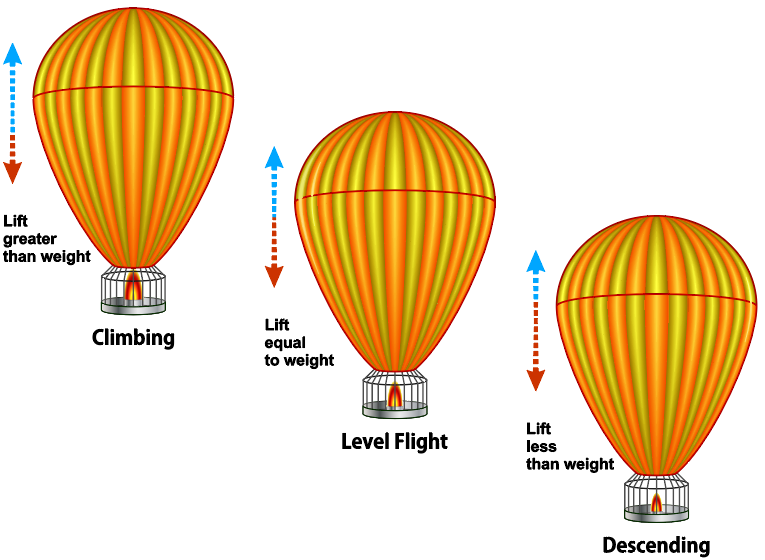So, what exactly is buoyancy? Buoyancy is the upward force exerted on an object placed in a fluid (gas or liquid). Even while on dry land buoyancy can affect you because you are in a fluid (air, which is a gas). It´s just that this force is so weak that it does not offset gravity, which is the downward force. A hot air balloon gives you a better idea of Archimedes' Principle on land as it rises, controlled by the same principles as when you rise up in the water by adding air to your BCD.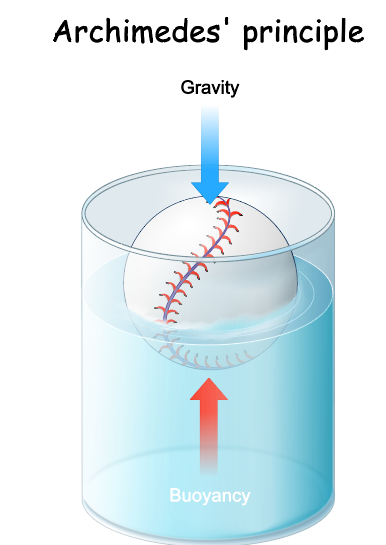Upwards force is the fluid (gas or liquid).

Downward force is gravity.

The amount of buoyancy – upward force- depends upon how much fluid the object is displacing, and the weight of the fluid displaced.

The denser the fluid, the more upward force (buoyancy) you get for a given volume of displacement.

Where buoyancy comes in play is the difference between the weight of the displacement and the weight of the objectWe just need to figure out the difference between the displacement of the object and the weight of the object and compensate for the difference by adding weight to sink the object (Kg) or displacing additional water, usually with a lift bag, to raise the object (Lt). In both cases, we always need to find out what is needed to make the object neutral.This all sounds so easy, but litres and kilograms are not compatible. Think apples and oranges, so you must convert one to match the other and this is where the confusion happens.

Your first step is changing Lt to Kg to find out the weight of the water displaced, exactly as it is stated in the wording at the end of the Archimedes principle.

The work starts here:

It is important to understand that there are many methods for teaching this theory and I am using just one. If this does not work for you, look online for alternate explanations.

There are 2 parts. Determining the 3 variables (OWD) and then using them in the equation (MSD)

VARIABLESAnd then we can plot out what is required from the physics question by using the following equation

EQUATION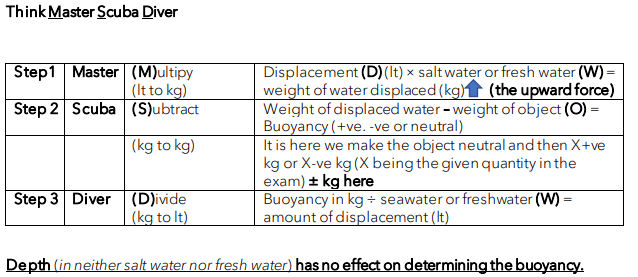So, let’s start with how the principle is worded and some of the confusion can be ironed out there.

Archimedes' principle states that the upward buoyant force that is exerted on a body immersed in a fluid, whether fully or partially submerged, is equal to the weight of the fluid that the body displaces.

Once you have determined the three variables (OWD) you can go onto the equation (MSD) and each step of MSD has a different purpose that we will go through individually

## 3 STEPS

STEP 1 IS TO FIND OUT THE WEIGHT OF THE WATER DISPLACED

(convert Lt – Kg) The upward force.Multiply (×) Lt to Kg

Displacement (Lt) × water constant (Kg) (salt or fresh) = weight of water displaced (Kg) (Upward force)

STEP 2 IS TO FIND OUT THE BUOYANCY OF THE OBJECT (+VE, -VE OR NEUTRAL)Subtract (-)

Weight of displacement (Kg) (upward force) – object weight (Kg) (downward force) = buoyancy.

• This finds the difference between the displacement of the object and the weight of the object

• This step is important to ascertain what type of buoyancy the object has. (+ve, -ve or neutral)

• From there we can work out what will make it neutrally buoyant.

• If it is positively buoyant you only need to add kg and the equation stops here as you do not need to displace air.

• Many questions stating that an object is neutrally buoyant will actually start here after the second step.

• Here is also where you add any kg to make it Xkg +ve or Xkg -ve. (Kg to Kg), we will do extra questions at the end to help out here.

STEP 3 IS TO FIND OUT THE DISPLACEMENT REQUIRED TO LIFT AN OBJECT (convert kg – lt)Divide (÷)

Buoyant weight of the object (ignore any +ve or -ve signs, they are only indicators) ÷ water constant = displacement required to lift the object.

• Only for lifting the object. If you are sinking the object, you do not need to know displacement.

• Neutral buoyancy is all you need for moving the object (upward force equals downward force)

• Be sure that you have made any adjustments for positive kg (previous step) if needed before completing this step.

• ONLY TO BE USED FOR DISPLACEMENT. (lifting) Think – DIVIDE TO DISPLACE

• Displacement is always in litres.

Put simply!

Step 1 Multiply; find the weight of displacement of water. (lt – kg)

Step 2 Subtract; is it +ve, -ve or neutral?

What adjustments do we need to make? (kg - kg)

Step 3 Divide; divide for displacement (kg – lt)

Ex 1 You Plan to recover a 150kg outboard motor in seawater that displaces 60 litres and lies at 30m. How much air must you put in a lifting device to make the motor neutrally buoyant?*weight of the object is More than the weight of the water displaced and therefore it sinks (-ve)

**Question only requires neutral buoyancy, so the amount of downward force is equal to the upward force.

The object is 88.20kg negative (downward force) Therefore 88.20kg positive is required to make the object neutral. Then we convert to litres. Remember, divide to displace.

88.20kg ÷ 1.03kg = 85.6 Lt

Ex2 You must sink an object that weighs 50 kg and displaces 300 Litres into fresh water, how much lead do you need to affix to the object to make it 10kg negative on the bottom?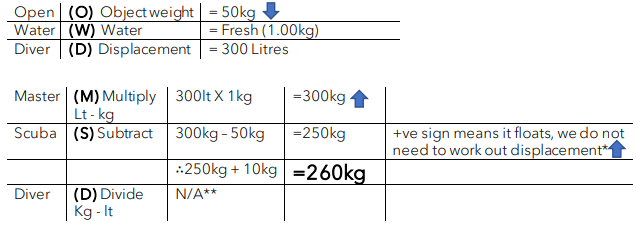* Weight of object is less than the weight of the water displaced and therefore floats (+ve)

**We first need to make the object neutral and then adjust for the extra 10 kg negative.

*** Because we are sinking the object, we do not need the third step. Remember Divide is only for Displace (kg-lt)

The object is 250kg positive (upward force) Therefore, 250kg negative (downward force) weight to make it neutral + 10kg negative (downward force) to make 10kg negatively buoyant.

250kg + 10kg = 260kg

Ex 3 An object weights 237kg and displaces 123litres of water. How much additional seawater do you need to displace to give the object 40kg positive buoyancy? (Round to closest litre)*weight of the object is More than the weight of the water displaced; therefore, it sinks (-ve)

**We first need to make the object neutral and then adjust for the extra 40kg positive.

*** Now we need to work out how many litres are displaced to raise the object to 40kg positive. Remember to divide to displace.

The object is 110.31kg negative (downward force) Therefore, 110.31kg positive (upward force) weight to make it neutral + 40kg positive (upward force) to make 40kg positively buoyant.

110.31kg + 40kg = 150.31kg

Then divide to determine how much water needs to be displaced

150.31kg ÷ 1.03kg = 146 lt (round to closest decimal point)

Ex 4 If an object weighing 150 kilograms pounds is neutrally buoyant in salt water, what is the volume of the water the object displaces?* We already know that the object is neutral and is in Kg so we do not need to do the first 2 steps

** We only need to know the displacement so Divide to Displace

150 ÷1.03 = 146 Lt

Below you can find links to both quizzes to test yourselves

Test yourselves on ArchimedesTest one is saltwater vs freshwaterTest two is the more complicated equations

## And to all the examsWater, Heat, Light, Sound, and Gases ExamArchimedes' Exam part 1Archimedes' Exam Part 2Under Pressure ExamBoyles' Law Exam Part 1

Single Level Depth ChangesBoyles' Law Exam Part 2

Multi Level Depth ChangesCharles' Law ExamHenrys' Law ExamDaltons' Law Exam